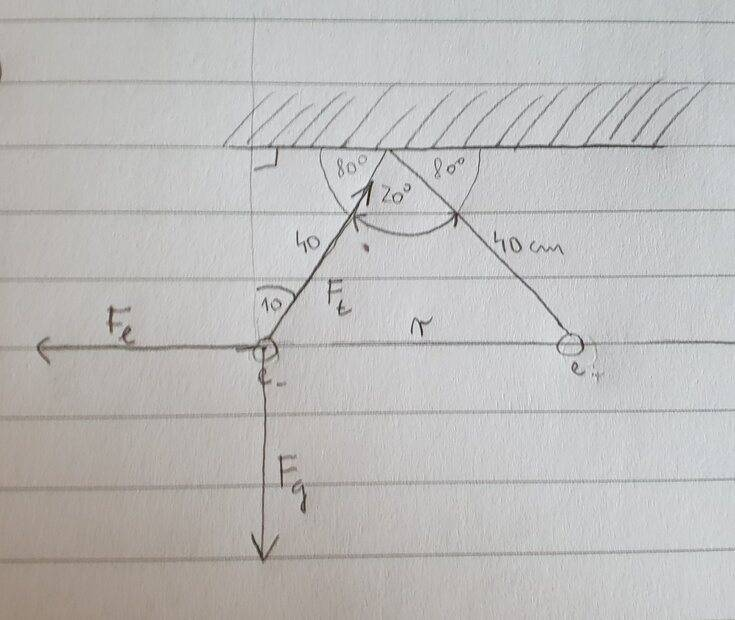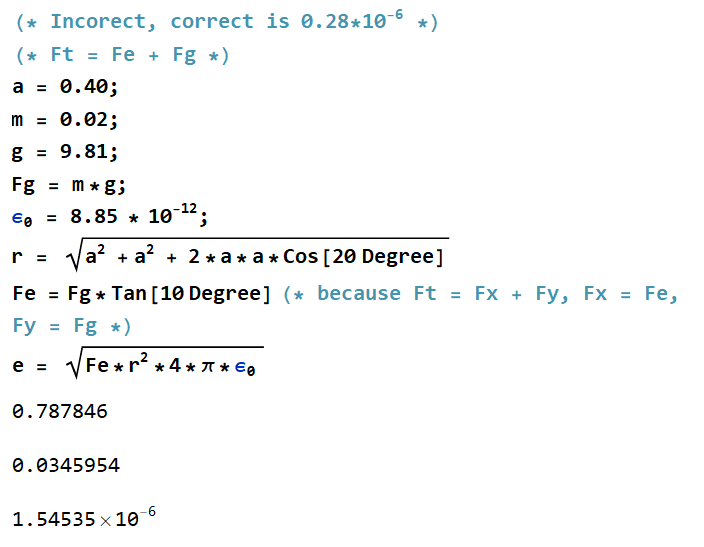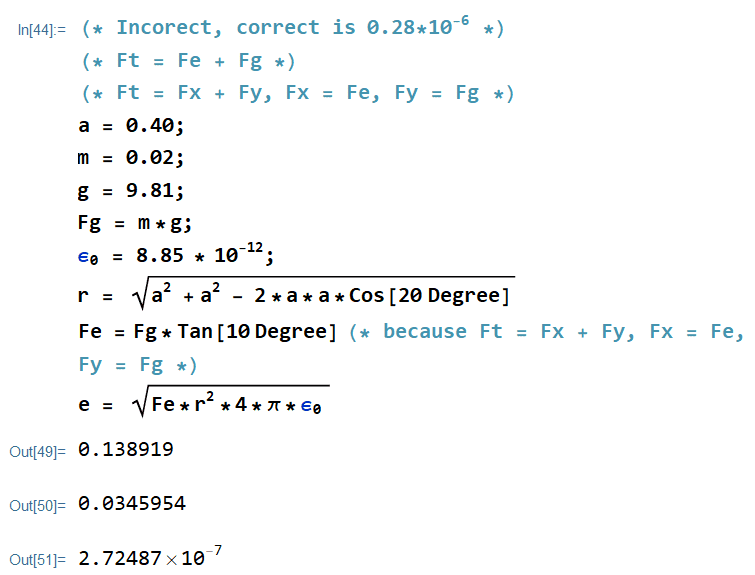# [Electrical force] 2 balls hanging from ceiling, angled

• sea333
In summary, the electric force is equal to the horizontal component of the tension, the weight is equal to the vertical component of the tension, and T=\sqrt{(mg)^2+F_e^2}.

#### sea333

Homework Statement
2 metal balls hang from a ceiling on a thread(see picture a.) ). After the balls are charged with the same charge both thread diverge to an angle 20 degrees.(see picture b.) )
What charge is used to charge each ball.
weight of each ball = 0.02 kg
Relevant Equations
F = (1/(4*Pi*eps0))*(e*e)/r^2#### Attachments

Your expression ##F_e=mg\tan(10^o)## is incorrect. The electric force is equal to the horizontal component of the tension, the weight is equal to the vertical component of the tension and ##T=\sqrt{(mg)^2+F_e^2}.##

kuruman said:
Your expression ##F_e=mg\tan(10^o)## is incorrect. The electric force is equal to the horizontal component of the tension, the weight is equal to the vertical component of the tension and ##T=\sqrt{(mg)^2+F_e^2}.##
ok but neither the Tension nor Fe are known, and we need Fe ?

Write down two equations balancing forces in the horizontal and vertical directions separately and see where you can go from there.

kuruman said:
Write down two equations balancing forces in the horizontal and vertical directions separately and see where you can go from there.
horizontal: Fx - Fe = 0 -> Fe = Fx
vertical: Fg - Fy = 0 -> Fg = Fy

sea333 said:
horizontal: Fx - Fe = 0 -> Fe = Fx
vertical: Fg - Fy = 0 -> Fg = Fy
This is correct but doesn't get you very far. You need to bring in the given quantities. Please use symbols like m and q and so on, not numbers. The numbers will be substituted in the final expression for q once you have it.

kuruman said:
This is correct but doesn't get you very far. You need to bring in the given quantities. Please use symbols like m and q and so on, not numbers. The numbers will be substituted in the final expression for q once you have it.
Fe = Fx
Fg = Fy
Fx = (1/(4*PI*Eps0))*(q*q/r^2)
Fy = mg

Right. But you have not brought in the information that the string makes a 10o angle with respect to the vertical. How would you go about doing that?

kuruman said:
Right. But you have not brought in the information that the string makes a 10o angle with respect to the vertical. How would you go about doing that?
that is cosinus -> T = mg / Cos10

Correct. What can you say about the horizontal direction?

this
Fx - Fe = 0
Fx = (1/(4*PI*Eps0))*(q*q/r^2)

And how is Fx related to T?

Fx/T = sin 10 -> Fx = Fe = T*sin10

OK. You have two equations one in the vertical direction and one in the horizontal direction involving T, mg and the electrical force. Can you write them down, one below the other?

T = mg / Cos10
Fx = Fe = T*sin10

Into the bottom equitation I can insert equation for T and get q which is the only unknown?

Please show me how you are going to do this.

q*q/(4*PI*Eps0*r^2) = (m*g / Cos10) * Sin10

And then just move everything except q on the right side

This looks easy, but why was my attempt flawed with Tan10 ?

sea333 said:
This looks easy, but why was my attempt flawed with Tan10 ?
I explained that in post #2.

sea333 said:
This looks easy, but why was my attempt flawed with Tan10 ?
That was fine, but you have a wrong sign in your use of the cosine rule to find r. Easier would have been ##r=a\sin(10°)##.

Edit: see correction in post #27.

Last edited:
haruspex said:
That was fine, but you have a wrong sign in your use of the cosine rule to find r. Easier would have been ##r=a\sin(10°)##.
When I changed the sign now result looks correct.
I don't think you can get r in such a way because angle 10 degrees is not adjacent to r

kuruman said:
I explained that in post #2.
It looks like the Tan approach was correct

correct solutionsea333 said:
When I changed the sign now result looks correct.
I don't think you can get r in such a way because angle 10 degrees is not adjacent to r
Sorry, I meant ##r=2a\sin(10°)##.

haruspex said:
Sorry, I meant ##r=2a\sin(10°)##.
I still don't see how this is correct as angle 10 is not adjacent to r

sea333 said:
I still don't see how this is correct as angle 10 is not adjacent to r
You have found that ##r=\sqrt{2a^2-2a^2\cos^2(20^o)}=\sqrt{2a^2(1-\cos(20^0))}##.
There is a trig identity that says
##\cos(2\theta)=\cos^2(\theta)-\sin^2(\theta)##
so that ##1-\cos(20^0)=1-\cos^2(10^o)+\sin^2(10^o)=2\sin^2(10^o)##. What do you get when you substitute in the expression for ##r##?

Another way to look at it is to break the isosceles triangle to two right triangles of base ##r/2## and hypotenuse ##a##. How is ##r/2## related to ##a##?

sqrt(4a^2sin^2(10)) = 2*a*sin10

How do you get from 1 - cos^2(10) - sin^2(10) to 2 sin^2(10) ?

sea333 said:
I still don't see how this is correct as angle 10 is not adjacent to r
Draw a vertical line down from the apex and consider the two triangles produced.

I don't think I will need these advance techniques in my exercises

sea333 said:
I don't think I will need these advance techniques in my exercises
Dropping a perpendicular from the apex of an isosceles triangle to create two congruent right angled triangles is pretty basic geometry; less advanced, even, than the cosine rule.

haruspex said:
Dropping a perpendicular from the apex of an isosceles triangle to create two congruent right angled triangles is pretty basic geometry; less advanced, even, than the cosine rule.
I have checked this and I get r = 2a*sin10

sea333 said:
I have checked this and I get r = 2a*sin10
Yes, as in my correction in post #27.

•sea333